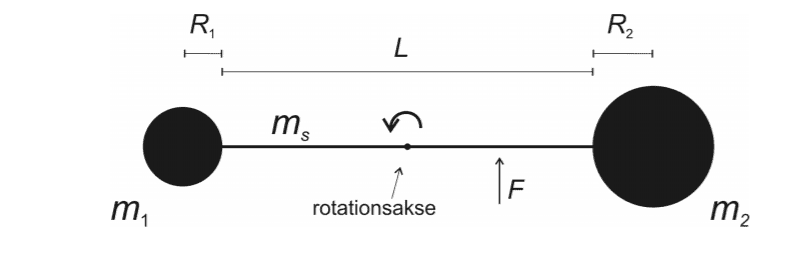# Rotational inertia - globes connected by a thin rod

Homework Statement:
Finding the rotational inertia
Relevant Equations:
I=∑m*r^2
Problem Statement: Finding the rotational inertia
Relevant Equations: I=∑m*r^2

A rigid body of 2 massive globes with homogenous mass distribution and a thin rod is connecting the 2 globes. The globes has radius R1 = 0.18 m and R2 =0.28 m and masses m1=193 kg and m2=726 kg. The thin rod has the mass ms=10kg and the length L=0.88 m. THe body can rotate frictionless around the rotation axis in the middle of the thin rod.

a) Calculate the the body's overall inertiamoment and show that the inertiamoment is I=476 kgm^2

my calculations are m1*(1/2*L+r1)^2+m2*(1/2*L+r2)^2 and i doubt if this is even correct and cant tell how the inertiamoment of the rod would look. Ignore the F(force)## Answers and Replies

Doc Al
Mentor
Hint: Don't treat the spheres as if they were point masses. (Think parallel axis theorem.)

haruspex
Science Advisor
Homework Helper
Gold Member
2020 Award
cant tell how the inertiamoment of the rod would look
You should have a standard formula for the MoI of a uniform stick about its centre. Similarly for a uniform solid sphere.

okay so for a solid sphere i use 2/5*m*r^2 and for the rod it is 1/12*m*L^2?

Doc Al
Mentor
okay so for a solid sphere i use 2/5*m*r^2 and for the rod it is 1/12*m*L^2?
Yes, about their respective centers.

Okay so you use the formula I=Icm+Md^2 and therefore:
2/5*m
1*r1^2+m1*(r1+1/2*L)^2 + 2/5*m2*r2^2+m2*(-r2-1/2*L)^2 + 1/12*m3*L+m3*1

haruspex
Science Advisor
Homework Helper
Gold Member
2020 Award
m3*1
Where does this term come from?
The rest is fine.

Where does this term come from?
The rest is fine.
honestly i am
Where does this term come from?
The rest is fine.

I am not sure how to calculate the rod when the rotation axis is right on the middle of it

haruspex
Science Advisor
Homework Helper
Gold Member
2020 Award
I am not sure how to calculate the rod when the rotation axis is right on the middle of it
That's what the ##\frac 1{12}mL^2## formula gives you. There is nothing to add in this case.

•naji0044